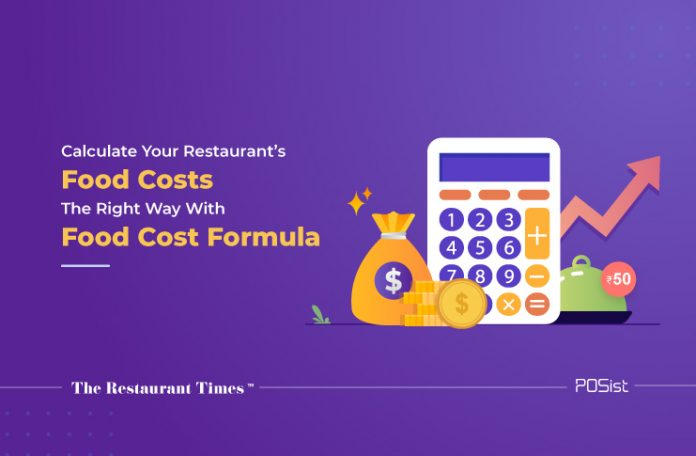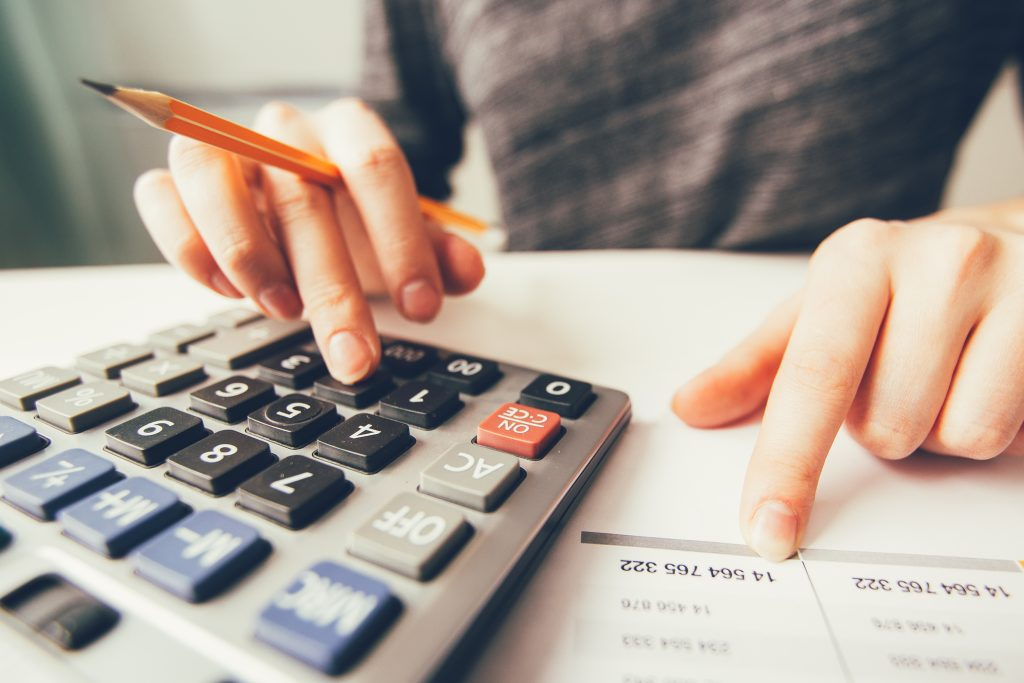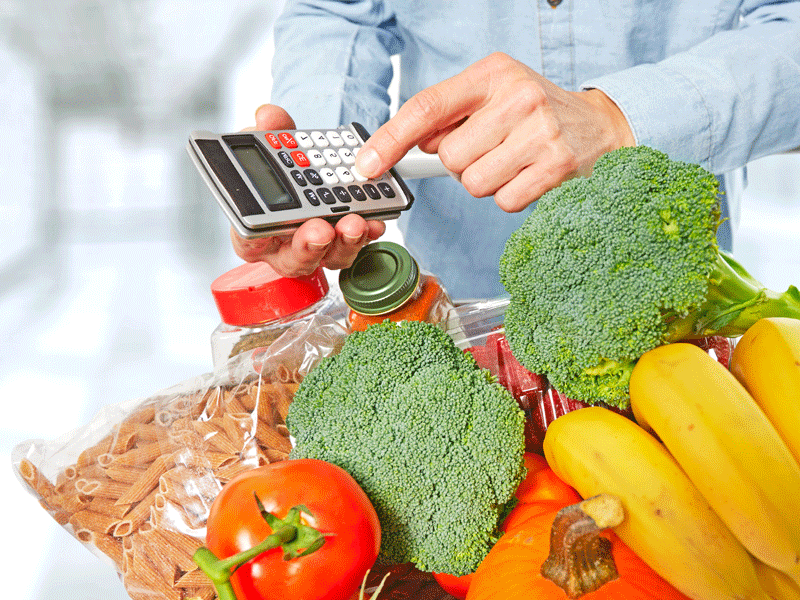# How Restaurants In Saudi Arabia Can Use Food Cost Formula To Manage InventoryAll restaurateurs in Saudi Arabia must calculate their food costs and make appropriate changes in the menu pricing to optimize the overall costs and decrease wastage. However, calculating the food cost isn’t an easy task. Often, most restaurant owners are unaware of how to use the correct food cost formula. In this article, we will explain the importance of food cost formula and how restaurants in Saudi Arabia can calculate food costs using the correct method.

## Importance Of Food Cost Formula

Food cost is the ratio between the raw material costs incurred in making a dish and the total revenue a restaurant generates. Most of the time, beverage costs are not included and counted separately. In percentage terms, the food cost is primarily a restaurant’s total food purchase, divided by the total sales.

Calculating the restaurant food costs greatly helps in maintaining a healthy business. However, figuring it with the help of a set of proper food cost formulae can help you achieve better accuracy, and reduce overall expenses. These are some of the reasons why calculating food costs is essential.

• Food cost determines a restaurant’s profitability. Without understanding the prices of various items, and their menu costs at different periods, it will be challenging for restaurants to run a highly profitable business. Analyzing the areas that require more adjustments and changes will help you garner more profit.
• Lower food cost ultimately generates higher profit and helps you to price your menu items correctly.

### The Correct Formula For Calculating Food Costs

Before understanding how to calculate the food cost of your restaurant, it is firstly important to understand the two types of food costs;#### Plate Cost

Plate cost is the amount you receive after dividing the value incurred while preparing a dish, divided by the selling price of the meal. To find the percentage, multiply this amount with 100.

Every restaurant must understand how much each plate, each item, or each drink costs them. Here’s the formula for calculating the plate cost:

The cost incurred while preparing a dish/the final selling price =  the plate cost

For instance, a single falafel costs \$2.00 to prepare/ and the selling price is \$8.00 = \$0.25 is your food cost.

The food cost percentage : 0.25 X 100= 25%

Therefore, the plate cost per falafel is 25%.

#### Period Cost

Period cost is slightly complicated, but it is essential for restaurants to keep a tab on this cost as well. Period food cost is essentially the cost incurred on food by a customer, in either a month or a quarter. However, if you want to determine the period food cost, you must know the COGS (Cost of Goods Sold) formula.

Period costs can determine the costs incurred over a period of time for any category in your restaurant.

The Formula for calculating COGS is:

Beginning Inventory + New Inventory Purchased – Ending Inventory = Total Food Usage in a particular period.

Once you gain the total amount, you can find the Cost Of Goods Sold by this formula :

Total Food Usage/Total Food Sales = COGS.

To calculate the COGS in percentage terms, multiply it with 100:

COGS X 100= COGS%

The period cost can determine food cost, beverage costs, and merchandise cost.

When using the COGS formula to calculate the food cost, ensure that you compare the right categories of inventory to the appropriate category sales. Make sure that you are comparing the right inventory and purchase period to the correct sales period.For instance, if you want to calculate the period cost for the month of July, you need to do the following:

Food beginning Inventory(for July) + Food purchases(in July) – Ending Inventory(at the end of the month) = Total food usage for the month of July

Once you have a particular month’s food usage, you can apply the COGS formula to understand the cost of the goods.

Food usage in July / Food sales in July = COGS

Multiply it by 100 to get the COGS amount in percentage values.

Once you have the food cost in place, you can bring in all the necessary changes to bolster your restaurant’s sales. By understanding the most selling and least selling menu items, you can apply the right menu engineering techniques and replace the least selling items with high-profit margin items. This will also help in reducing the overall operating expenses.

Follow the food cost formula in Saudi Arabia and apply it appropriately to keep your cash registers overflowing!